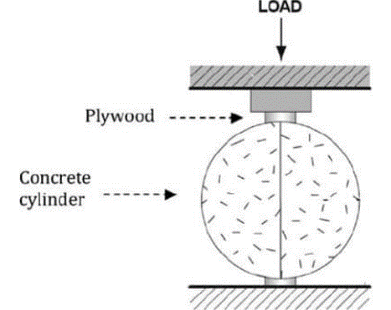# Tensile strength determination of concrete (splitting)

## Introduction:

The sample of concrete enjoys compression whereas when it comes towards tension it has a brittle and weak nature. Therefore, capacity of maximum load and the strength of the sample is determined in terms of tension also it is not preferable to apply a direct tension load.

## Related theory:

The nature of concrete and its behavior is highly effective in the structural design of various elements. Its reaction towards tension is highly observed as it leads to abrupt rupture because of its brittle nature which makes the structure at high risk.

This test is basically the determination of the max load that it can carry in tension. For this a certain setup is adopted by using the bearing plates and bars. The load is to be applied at a constant loading rate. The load application is based on the sample size as follows:

The machine used for this test and its assembly is as follows:

Figure 1 tensile strength (splitting) testing machine

### Bearing Bars:

A bearing bar is adopted when largest dimension of any of its surface is less in comparison of machine. Its width is 50mm usually and its application is such that the load application is such that it has uniform distribution entirely.

###These are used for the proper and periodic load distribution over the surface. Two of these are used with size 25mm width, 3.2mm thickness and length extended than the sample adopted for the test. These are provided based on its need.as shown in the figure.

This test is very important in the concrete structure depending on its life and stability.

## Standards:

• ASTM C496-11
• ASTMC39-14 (specimen)
• ASTM C-796
• IS 58161999

## Apparatus:

• Diamond core tip (machine)
• Machine for compression testing
• Set of calipers
• Gypsum pastes with high strength
• Permanent markers
• A ruler
• Sample of concrete properly prepared
• Bearing bars & strips

## Procedure:

1. concrete sample is already prepared and cured according to its specifications is adopted.
2. surface of the sample is observed if not even it is properly levelled by using gypsum to have uniform load distribution of the sample.
3. It is then positioned in the lading machine and observed the bearing plates are required or not. If required they are given.
4. loading is applied with a persistent rate depending on its standard.
5. This loading is removed when, cracks are detected in the sample observed with the maximum rupture value.
6. a ruler is applied to ascertain the crack length of the observed sample.
7. The final statement is reported for minimum of three samples and an average value for calculations are reported.

## Observations:

tensile strength (splitting),T (psi) = ———-

maximum load application through machine, P (lbs.) = ———-

samples average length, L (in.) = ———-

Diameter (sample), D (in.) = ———-

## Calculations:

splitting strength i.e. Tensile of the sample is determined as:

Sample #1 = ——- psi

Sample #2 = ——- psi

Splitting strength= (sample #1+sample #2)/2 = ——- psi

The concrete behavior, maximum load bearing capacity, its behavior towards the tension force is observed and commented.

## Precautions:

Following are the safety measures chosen:

1. safety glasses are observed as because of brittle and flaky nature.
2. The Sample is to be properly cured.
3. Ruler is used for the crack length determination.
4. The Load calibrations have to be determined accurately.
##### Related Posts## To determine the workability of concrete mix of given proportion by compaction factor test.

Compacting factor test is performed using guidelines from past researches. However, BS 1881-103 has detailed the guidelines for…## Determination of the flexural strength of concrete

Introduction: This test is used to determine the deflection produce in the slab made up of concrete due…## To determine the fineness modulus of given fine and coarse aggregates.

Introduction: Fineness modulus is the average particle size present in any mixture. In a concrete mixture it determines…